×

Search anything:

# Skew Heap

#### Data Structures heapGet this book -> Problems on Array: For Interviews and Competitive Programming

In this article, we will discuss about skew heap. Skew heap is a special variant of Leftist heap. Leftist heap is in turn, a variant of binary heap.
We will first give an overview of binary heaps, then discuss leftist heaps and finally talk about skew heaps.

## Binary Heaps

Binary heaps are fundamentally, binary trees with some constraints on node values. Binary trees can have a maximum of 2 child nodes. A single child must always be the left child of it's parent. Binary heaps are of 2 types: Min heaps and Max heaps. In min heaps, the value of the root node must be smaller than it's child node values. Similarly, in max heaps, the value of the root node must be greater than it's child node values.
With Binary heaps, operations like insert and delete are of the order O(logn). Merge operation is of the order O(nlogn).

## Leftist Heaps

A Leftist (min) Heap is a binary tree that satisfies the following conditions. If X is a node and L and R are its left and right children, then:

• X.value ≤ L.value
• X.value ≤ R.value
• Null path length of L ≥ Null path length of R,
where the null path length of a node is the shortest path from that node to a descendant with 0 or 1 child. If a node is null, its null path length is -1.
Leftist trees offer operations like insert, delete and merge in O(logn) time. A leftist heap attempts to maintain balance by unconditionally swapping all nodes in the merge path when merging two heaps.

## Skew Heaps

Skew heaps offer faster merge time as they are a special case of leftist trees. A skew heap is not structurally restricted. This can make the height of the tree non logarithmic. Skew heaps follow the basic properties of binary heaps i.e the root value is smaller than it's child nodes(min heap) or root value is greater than it's child nodes(max heap). Apart from this, another important property that must be followed is that operations like insert and delete are performed only using the merge operation in the background. We will see how these operations are rendered using the merge operation later in the article. Skew heaps, like leftist heaps offer a time complexity of O(logn) for insert, delete and merge operations. Please note that the time complexity of all these operations is amortized O(logn). Amortized time complexity means that there are a few operations which are more costly than others. The average of all the operations will be result in a time complexity of O(logn).

## Definition of Skew Heap

This is the recursive definition of skew heap:

• A heap with only one element is a skew heap.
• The result of merging two skew heaps S1 and S2 is also a skew heap.

# Operations

### Merging

Merge operation can be performed by following these steps recursively:

• Compare roots of two heaps; let P be the heap with the smaller root, and Q be the other heap. Let R be the resulting heap after merging.
• Let the root of R be the root of P (the smaller root). The left subtree of P becomes the right subtree of R.
• Now, compute R's left subtree by recursively merging P's right subtree with Q.

Alternatively, the following non-recursive steps can also be used to merge 2 skew heaps.

• Split the tree such that the left subtree becomes the one part. The right subtree becomes a new tree and is split just like the parent tree (left subtree becomes a new tree and right tree is split again). This splitting is done until the tree has either a left child or no children.
• Sort the resulting set of trees according to their root values in ascending order.
• While there are more trees left, recombine the trees starting from the last two.
• If the root of the second-to-last subtree has a left child, swap it to be the right child.
• Link the root of the last subtree as the left child of the second-to-last subtree.

Following is an example, for merging 2 skew heaps: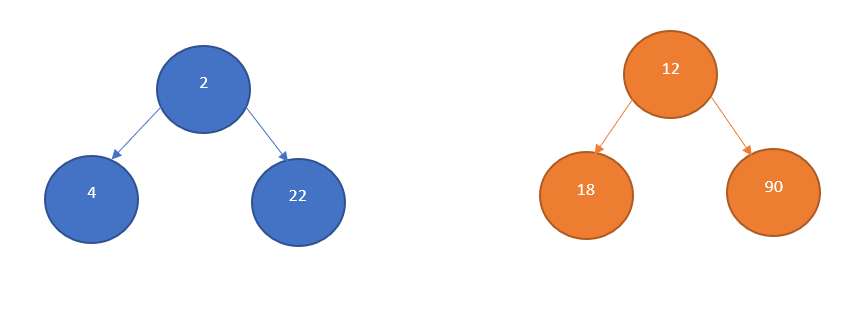After splitting both the trees, we get the following subtrees.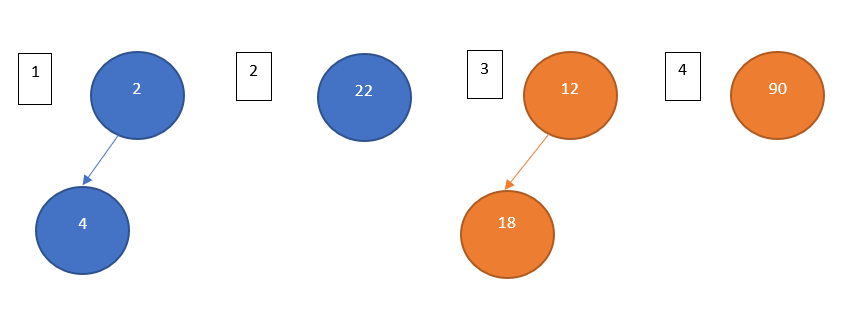All the subtrees are alloted numbers in the diagram above, sorting them in ascending order gives us this sequence: 1 < 3 < 2 < 4

Now, we start merging the subtrees starting from the last two in the list. Make sure that you maintain the min/ max heap at all times.
Merging subtree 2 and subtree 4: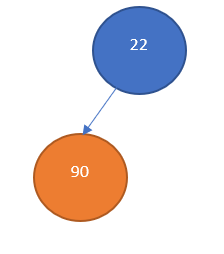Next, we merge this tree and subtree 3: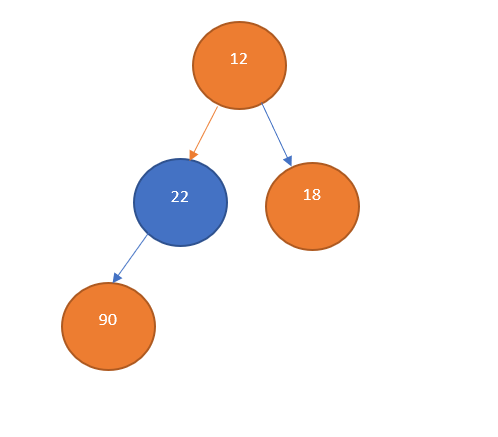Finally, we merge this tree and subtree 1 to get the final tree: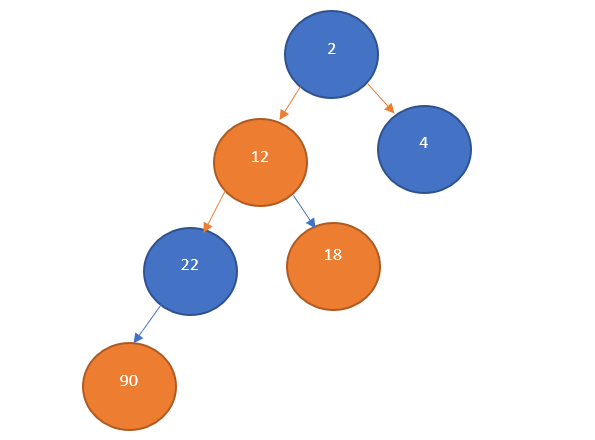Adding a new node to the skew heap is equivalent to the operation of merging a single node skew heap with the original skew heap.

### Removing a node

Removing a node can be accomplished by removing the root node and merging the left and right subtrees.

# Pseudo Code

• Merge
``````SkewHeap merge(SkewHeap s1,SkewHeap s2)
{
if(s1 == null)
return s2;
if(s2 == null)
return s1;
SkewHeap newRoot = null;
if(s1.data < s2.data)
{
newRoot = s1;
newRoot.right = s1.left;
newRoot.left = merge(p.right, s2);
}
else
merge (s2, s1);
return newRoot;
}
``````
``````void add(SkewHeap s, SkewHeap newNode)
{
merge(s, newNode);
}
``````
• Remove/Extract Min
``````SkewHeap remove(SkewHeap s)
{
if(s == null)
return s;
int res = s.value;
SkewHeap left = s.left;
SkewHeap right = s.right;
print("Minimum value is " + res);   //maximum in case of max heap
return merge(left, right);
}
``````

# Time complexity

As discussed earlier, merging 2 skew heaps requires O(logn) amortized time. Let's take a closer look at how this is obtained.

Merging a skew heap recursively using the naive technique, makes the smaller root as the new root and merges it's right subtree with the other subtree, keeping the left tree as before. This recursive idea can lead to a right heavy tree meaning that the resultant tree after multiple merge operations is skewed to the right. In the worst case, this can result in O(n) time complexity. To make sure that this does not happen and the tree is well balanced and offers a time complexity of O(logn), we add an extra step to the naive merging technique. After each recursive merge, we swap the left and right subtrees of the tree. This significantly affects the time complexity and balances the height of the tree resulting in O(logn) time complexity. We can observe this phenomenon in the recursive method discussed earlier in the article where the right subtree of the new tree is the left subtree of the smaller root value tree.